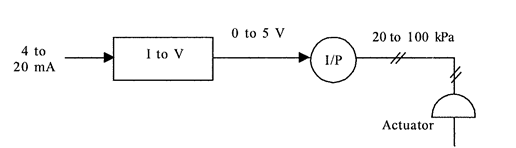# Assignment and lab | Electronic Engineering homework help

## Laplace Transform and the Analysis in the s-domain

1. Work Exercises 4, 20, 22, 27 and 37 from Chapter 14.
2. Work Exercises 11 and 16 from Chapter 15.
3. Save all the work in HW6_StudentID and upload. Show all work for full credit.

## Laplace Transform and Analysis in S-Domain

This week’s lab is based on the application of circuit analysis techniques to a capacitive circuit with Multisim. You will learn to utilize Multisim to perform the mesh analysis.

1. Watch video entitled “Week 6 – Oscillator Circuits in Multisim”
2. Design a Wein bridge Oscillator in Figure 15.39 in Multisim to generate a sinusoidal signal at frequency of 300Hz. Consider a capacitor of 1uF, resistor R = 5950Ω, R1 = 3Ω, Rf = 6.05Ω for the oscillator circuit.
1. Determine output voltage ‘Vo’ and run the simulation to plot the output of the oscillations at frequency of 300 Hz using an oscilloscope.
2. Increase the frequency to 500Hz, 800Hz and 1000Hz and plot the output of the oscillator at these multiple frequencies.
3. Take the screen capture of the oscilloscope readings for all the frequencies.
1. What is the requirement to generate oscillation in the circuit? Is the circuit stable or unstable?
2. What do you observe in the oscillations when the frequency is increased?
3. With increased frequency, did you observe the oscillations? If not what did you adjust to observe the oscillations?
4. Explore different practical applications of oscillator circuits and explain.
4. Create a new word document called “Lab6_StudentID.docx” with your GID substituted into the file name.
5. Save the simulation results from step 2 along with the measurements and screen captures. Make sure to answer the questions in step 6.

## Final Control

Work the problems below. To receive any credit, you must show all work. You may submit your work in a word processing document or in a pdf file. Graphic files are not acceptable submissions. Your file submission document should be entitled Week6AYourGID (replace YourGID with your specific GID).

1. An SCR with a 5V gate trigger level is used with a 12V zener diode, and the capacitor is 0.15uF. What value of R2 will give full control of the power to the load down to zero?
2. A stepping motor has 120 steps per revolution. Find the digital input rate that produces 10.2 rev/s.
3. What force is generated by a 85 kPa acting on a 25 cm2 area diaphragm?
4. Find the proper valve size in inches for pumping a liquid flow rate of 580 gal/min with a maximum pressure difference of 50 psi. The liquid specific gravity is 1.3.The SCR in Figure 1 below requires a 3 V trigger.  Using Multsim, design a system by which the gears are shifted when a CdS photocell resistance drops below 4kohms.

## Final Control Lab

1. The SCR in Figure 1 below requires a 3 V trigger.  Using Multsim, design a system by which the gears are shifted when a CdS photocell resistance drops below 4kohms.Figure 1

2. Using Multisim, design a system by which a control signal of 4 to 20 mA is converted into a force of 200 to 1000N.  Use a pneumatic actuator and specify the required diaphragm area if the pressure output is to be in the range of 20 to 100kPa.  An I/P converter is available that converts 0 to 5 V into 20 to 100 kPa.  A block diagraph of the system is shown below.  (Hint:  Use a differential amplifier.  You are only designing the circuit to interface into the I/P below)